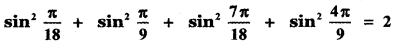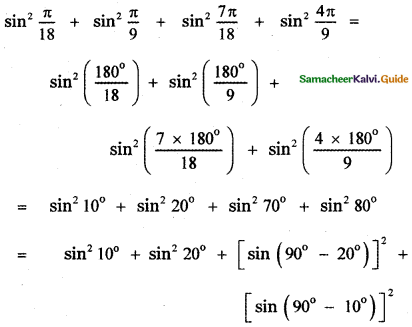Tamilnadu State Board New Syllabus Samacheer Kalvi 11th Maths Guide Pdf Chapter 3 Trigonometry Ex 3.3 Text Book Back Questions and Answers, Notes.

## Tamilnadu Samacheer Kalvi 11th Maths Solutions Chapter 3 Trigonometry Ex 3.3

Question 1.
Find the values of
(i) sin 480°
(ii) sin (-1110°)
(iii) cos 300°
(iv) tan (1050°)
(v) cot 660°
(vi) tan $$\left(\frac{19 \pi}{3}\right)$$
(vii) sin $$\left(\frac{-11 \pi}{3}\right)$$
(i) sin(480°) = sin(360° + 120°) = sin 120°
= sin(90° + 30°) = cos 30° = $$\sqrt{3}$$/2

(ii) sin(-1110°) = -sin(1110°)
= – sin (360° × 3 + 30°)
= -sin 30° = -1/2

(iii) cos(300°) = cos(270° + 30°) = sin 30° = 1/2

(iv) tan (1050°)
tan (1050°) = tan(12 × 90 – 30°)
= – tan30° = – $$\frac{1}{\sqrt{3}}$$

(v) cot 660°
cot 660° = cot (7 × 90 + 30°)
= – tan 30° = – $$\frac{1}{\sqrt{3}}$$

(vi) tan $$\left(\frac{19 \pi}{3}\right)$$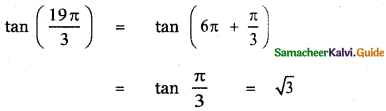(vii) sin $$\left(\frac{-11 \pi}{3}\right)$$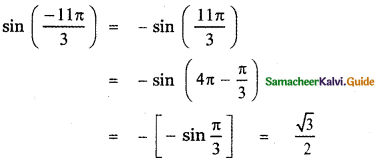Question 2.
$$\left(\frac{5}{7}, \frac{2 \sqrt{6}}{7}\right)$$ is a point on the terminal side of an angle θ in standard position. Determine the six trigonometric function values of angle θ.
Given $$\left(\frac{5}{7}, \frac{2 \sqrt{6}}{7}\right)$$ is a point on the terminal side of an angle θ in standard position.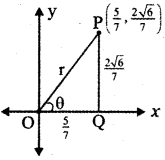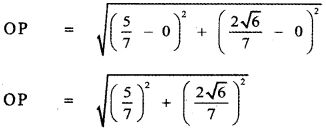Question 3.
Find the values of the other five trigonometric functions of the following
(i) cos θ = –$$\frac{1}{2}$$, θ lies in the III quadrant
(ii) cos θ = $$\frac{2}{3}$$, θ lies in the I quadrant
(iii) sin θ = –$$\frac{2}{3}$$, θ lies in the IV quadrant
(iv) tan θ = – 2, θ lies in the II quadrant
(v) sec θ = $$\frac{13}{5}$$, θ lies inthe IVquadrant
(i) cos θ = –$$\frac{1}{2}$$, θ lies in the III quadrant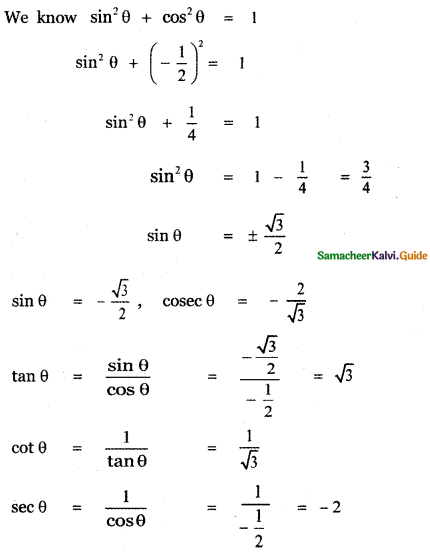(ii) cos θ = $$\frac{2}{3}$$, θ lies in the I quadrant
We know that cos2θ + sin2θ = 1
$$\left(\frac{2}{3}\right)^{2}$$ + sin2θ = 1
$$\frac{4}{9}$$ + sin2θ = 1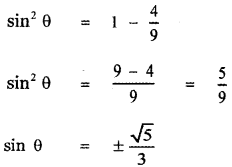Since θ lies in the I quadrant all trigonometric functions are positive.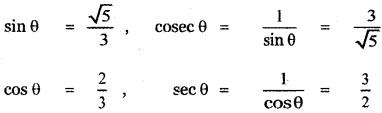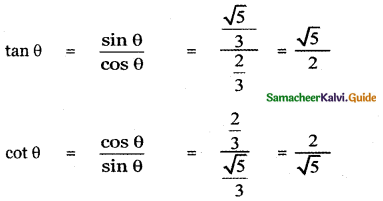(iii) sin θ = –$$\frac{2}{3}$$, θ lies in the IV quadrant
We know that cos2θ + sin2θ = 1
cos2θ + $$\left(-\frac{2}{3}\right)^{2}$$ = 1
cos2θ + $$\frac{4}{9}$$ = 1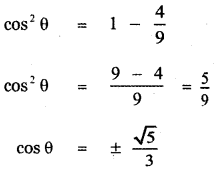Since θ lies in the fourth quadrant cos θ is positive.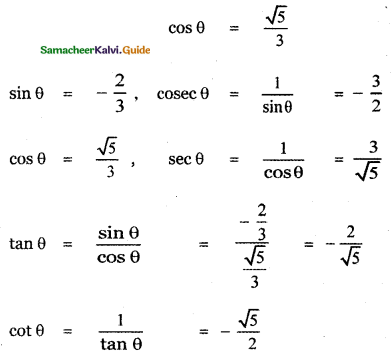(iv) tan θ = – 2, θ lies in the II quadrant
We know that sec2θ – tan2θ = 1
sec2θ – (-2)2 = 1
sec2θ – 4 = 1
sec2θ = 1 + 4 = 5
sec θ = ± √5
Since θ lies in the second quadrant sec θ is negative.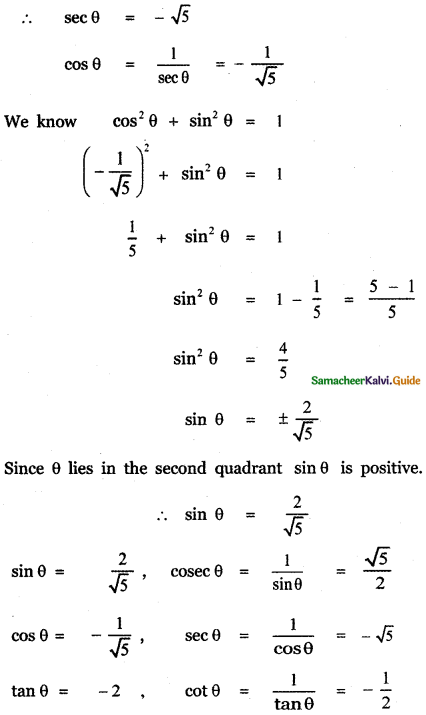(v) sec θ = $$\frac{13}{5}$$, θ lies inthe IVquadrant
We know that sec2θ – tan2θ = 1
$$\left(\frac{13}{5}\right)^{2}$$ – tan2θ = 1
$$\frac{169}{25}$$ – 1 = tan2θ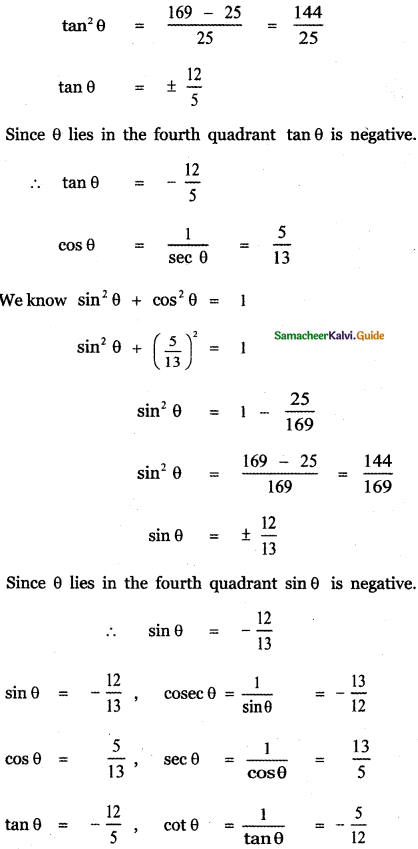Question 4.
Prove that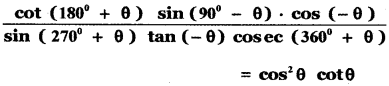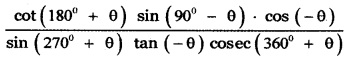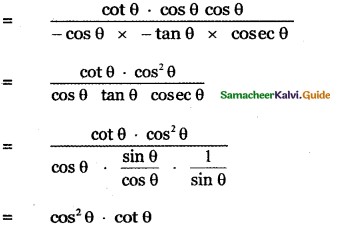Question 5.
Find all the angles between 0° and 360° which satisfy the equation sin2θ = $$\frac{3}{4}$$
sin2θ = $$\frac{3}{4}$$ ⇒ sin θ = ± $$\frac{\sqrt{3}}{2}$$
sin 60° = $$\frac{\sqrt{3}}{2}$$
sin 120° = sin (180° – 60°)
= sin 60° = $$\frac{\sqrt{3}}{2}$$
∴ θ = 60° and 120°Question 6.
Show that